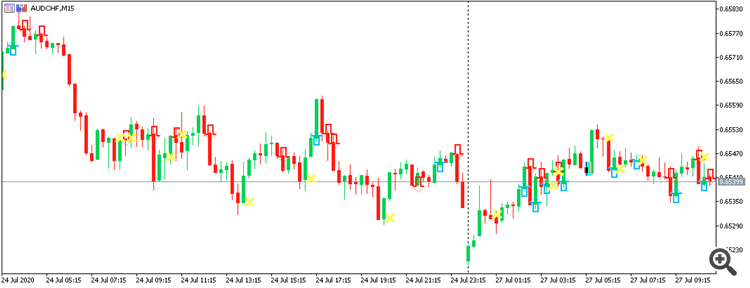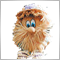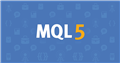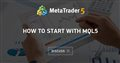# how to expose hidden arrow objects from custom indicator in chart's objects list212

I have an indicator that draws arrows on the chart as seen in the image below:I tried searching for the arrow objects in ' Object List'  but there were no objects there. I also checked ' Object List>List All'  menu. I guess the way that the indicator is written has hid them from the chart. How can I expose these objects from the indicator to the chart?

Here are some relevant bits of my indicator:

```//--- indicator settings
#property indicator_chart_window
#property indicator_buffers 6
#property indicator_plots 6

#property indicator_type1 DRAW_ARROW
#property indicator_width1 5
#property indicator_color1 0xFFAA00

#property indicator_type2 DRAW_ARROW
#property indicator_width2 5
#property indicator_color2 0x0000FF
#property indicator_label2 "Sell"

#property indicator_type3 DRAW_ARROW
#property indicator_width3 5
#property indicator_color3 0xFFAA00

#property indicator_type4 DRAW_ARROW
#property indicator_width4 5
#property indicator_color4 0x0000FF
#property indicator_label4 "Sell"

#property indicator_type5 DRAW_ARROW
#property indicator_width5 5
#property indicator_color5 0x00FFFB

#property indicator_type6 DRAW_ARROW
#property indicator_width6 5
#property indicator_color6 0x00FFFB
#property indicator_label6 "CLOSE SELL"

//--- indicator buffers
double Buffer1[];
double Buffer2[];
double Buffer3[];
double Buffer4[];
double Buffer5[];
double Buffer6[];

double myPoint; //initialized in OnInit
int MA_handle;
double MA[];
int MA_handle2;
double MA2[];
int MACD_handle;
double MACD_Signal[];
int MA_handle3;
double MA3[];
double Close[];
int MA_handle4;
double MA4[];
int MA_handle5;
double MA5[];
int MA_handle6;
double MA6[];
double Open[];

.
.
.

int OnInit()
{
SetIndexBuffer(0, Buffer1);
PlotIndexSetDouble(0, PLOT_EMPTY_VALUE, EMPTY_VALUE);
PlotIndexSetInteger(0, PLOT_ARROW, 241);
SetIndexBuffer(1, Buffer2);
PlotIndexSetDouble(1, PLOT_EMPTY_VALUE, EMPTY_VALUE);
PlotIndexSetInteger(1, PLOT_ARROW, 242);
SetIndexBuffer(2, Buffer3);
PlotIndexSetDouble(2, PLOT_EMPTY_VALUE, EMPTY_VALUE);
PlotIndexSetInteger(2, PLOT_ARROW, 241);
SetIndexBuffer(3, Buffer4);
PlotIndexSetDouble(3, PLOT_EMPTY_VALUE, EMPTY_VALUE);
PlotIndexSetInteger(3, PLOT_ARROW, 242);
SetIndexBuffer(4, Buffer5);
PlotIndexSetDouble(4, PLOT_EMPTY_VALUE, EMPTY_VALUE);
PlotIndexSetInteger(4, PLOT_ARROW, 251);
SetIndexBuffer(5, Buffer6);
PlotIndexSetDouble(5, PLOT_EMPTY_VALUE, EMPTY_VALUE);
PlotIndexSetInteger(5, PLOT_ARROW, 251);
//initialize myPoint
myPoint = Point();
if(Digits() == 5 || Digits() == 3)
{
myPoint *= 10;
}
MA_handle = iMA(NULL, PERIOD_CURRENT, 1, 0, MODE_SMA, PRICE_CLOSE);
if(MA_handle < 0)
{
Print("The creation of iMA has failed: MA_handle=", INVALID_HANDLE);
Print("Runtime error = ", GetLastError());
return(INIT_FAILED);
}

.
.
.```Moderator
207274

The drawing style 'DRAW_ARROW' does not create graphic objects. Therefore, you cannot find these objects in the object list.212

The drawing style 'DRAW_ARROW' does not create graphic objects. Therefore, you cannot find these objects in the object list.

What should I use in place of 'DRAW_ARROW'?Moderator
207274

Jackery :

What should I use in place of 'DRAW_ARROW'?

It is not at all clear why you want to see objects in the general list?

Just keep using the 'DRAW_ARROW' style - this is the most correct approach.212

It is not at all clear why you want to see objects in the general list?

Just keep using the 'DRAW_ARROW' style - this is the most correct approach.

I want to write an EA that opens a trade when an arrow is drawn on the index 1 candlestick. The idea is once it's drawn on the chart I should be able to access the object in my EA code. There might be a way to access them directly from the indicator without doing that, but I'm not sure how that could be done.Moderator
207274

Jackery :

I want to write an EA that opens a trade when an arrow is drawn on the index 1 candlestick. The idea is once it's drawn on the chart I can easily access the object in my EA code. There might be a way to access them directly from the indicator without doing that, but I'm not sure how that could be done.

You just need in an advisor: create an indicator handle (once in OnInit ()) and then receive data from the indicator buffer.

Everything is very simple. Read help: iCustom . See examples: An example of working with the ZigZag indicator.Documentation on MQL5: Technical Indicators / iCustom
• www.mql5.com
[in]  Custom indicator name. If the name starts with the reverse slash '\', the EX5 indicator file is searched for relative to the MQL5\Indicators indicator root directory. Thus, when calling FirstIndicator"...), the indicator is downloaded as MQL5\Indicators\FirstIndicator.ex5. If the path contains no file, the error 4802...212

You just need in an advisor: create an indicator handle (once in OnInit ()) and then receive data from the indicator buffer.

Everything is very simple. Read help: iCustom . See examples: An example of working with the ZigZag indicator.

To be honest, I am don't fully understand how to implement the example to get the arrow object. I think I've been able to figure out how to get the  moving averages but not the arrow object. Below is the code iCustom EA I've written:

```double      MA[];
int         MA_handle;

//+------------------------------------------------------------------+
//| Expert initialization function                                   |
//+------------------------------------------------------------------+
int OnInit()
{

MA_handle=iCustom(NULL,PERIOD_M1,"R_IND");

return(0);
}
//+------------------------------------------------------------------+
//| Expert tick function                                             |
//+------------------------------------------------------------------+
void OnTick()
{

CopyBuffer(MA_handle,0,0,3,MA);

ArraySetAsSeries(MA,true);

Print( "h MA: "+MA);

}```Moderator
207274

First, create and design your indicator correctly - at least the indicator should have a NAME and EXTENSION. Publishing a PIECE of code without a HAT, without a NAME and without an EXTENSION is bad form in programming.

An example of creating an indicator:

### Example of creating an indicator based on iATR• 2020.07.05
• www.mql5.com
This thread discusses MQL5 code examples. There will be examples of how to get data from indicators, how to program advisors...212

First, create and design your indicator correctly - at least the indicator should have a NAME and EXTENSION. Publishing a PIECE of code without a HAT, without a NAME and without an EXTENSION is bad form in programming.

An example of creating an indicator:

### Example of creating an indicator based on iATR

Here is the complete working indicator:

```//+------------------------------------------------------------------+
//|                                             MyScalpIndicator.mq5 |
//|                        Copyright 2019, MetaQuotes Software Corp. |
//|                                             https://www.mql5.com |
//+------------------------------------------------------------------+
#property version   "1.00"
#property description ""

//--- indicator settings
#property indicator_chart_window
#property indicator_buffers 2
#property indicator_plots 2

#property indicator_type1 DRAW_ARROW
#property indicator_width1 5
#property indicator_color1 0xFFAA00

#property indicator_type2 DRAW_ARROW
#property indicator_width2 5
#property indicator_color2 0x0000FF
#property indicator_label2 "Sell"

//--- indicator buffers
double Buffer1[];
double Buffer2[];

double myPoint; //initialized in OnInit
int MA_handle;
double MA[];
int MA_handle2;
double MA2[];
double Open[];

{
if(type == "print")
Print(message);
else if(type == "error")
{
Print(type+" | SPON SCALP BOSS @ "+Symbol()+","+IntegerToString(Period())+" | "+message);
}
else if(type == "order")
{
}
else if(type == "modify")
{
}
else if(type == "indicator")
{
}
}

//+------------------------------------------------------------------+
//| Custom indicator initialization function                         |
//+------------------------------------------------------------------+
int OnInit()
{
SetIndexBuffer(0, Buffer1);
PlotIndexSetDouble(0, PLOT_EMPTY_VALUE, EMPTY_VALUE);
PlotIndexSetInteger(0, PLOT_ARROW, 241);
SetIndexBuffer(1, Buffer2);
PlotIndexSetDouble(1, PLOT_EMPTY_VALUE, EMPTY_VALUE);
PlotIndexSetInteger(1, PLOT_ARROW, 242);
//initialize myPoint
myPoint = Point();
if(Digits() == 5 || Digits() == 3)
{
myPoint *= 10;
}
MA_handle = iMA(NULL, 0, 3, 0, MODE_SMA, PRICE_CLOSE);
if(MA_handle < 0)
{
Print("The creation of iMA has failed: MA_handle=", INVALID_HANDLE);
Print("Runtime error = ", GetLastError());
return(INIT_FAILED);
}

MA_handle2 = iMA(NULL, 0, 10, 0, MODE_SMA, PRICE_CLOSE);
if(MA_handle2 < 0)
{
Print("The creation of iMA has failed: MA_handle2=", INVALID_HANDLE);
Print("Runtime error = ", GetLastError());
return(INIT_FAILED);
}

return(INIT_SUCCEEDED);
}

//+------------------------------------------------------------------+
//| Custom indicator iteration function                              |
//+------------------------------------------------------------------+
int OnCalculate(const int rates_total,
const int prev_calculated,
const datetime& time[],
const double& open[],
const double& high[],
const double& low[],
const double& close[],
const long& tick_volume[],
const long& volume[],
{
int limit = rates_total - prev_calculated;
//--- counting from 0 to rates_total
ArraySetAsSeries(Buffer1, true);
ArraySetAsSeries(Buffer2, true);
//--- initial zero
if(prev_calculated < 1)
{
ArrayInitialize(Buffer1, 0);
ArrayInitialize(Buffer2, 0);
}
else
limit++;
datetime TimeShift[];
datetime Time[];

if(BarsCalculated(MA_handle) <= 0)
return(0);
if(CopyBuffer(MA_handle, 0, 0, rates_total, MA) <= 0) return(rates_total);
ArraySetAsSeries(MA, true);
if(CopyTime(Symbol(), PERIOD_CURRENT, 0, rates_total, TimeShift) <= 0) return(rates_total);
ArraySetAsSeries(TimeShift, true);
if(BarsCalculated(MA_handle2) <= 0)
return(0);
if(CopyBuffer(MA_handle2, 0, 0, rates_total, MA2) <= 0) return(rates_total);
ArraySetAsSeries(MA2, true);
if(CopyOpen(Symbol(), 0, 0, rates_total, Open) <= 0) return(rates_total);
ArraySetAsSeries(Open, true);
if(CopyTime(Symbol(), Period(), 0, rates_total, Time) <= 0) return(rates_total);
ArraySetAsSeries(Time, true);
//--- main loop
for(int i = limit-1; i >= 0; i--)
{
if (i >= MathMin(5000-1, rates_total-1-50)) continue; //omit some old rates to prevent "Array out of range" or slow calculation

int barshift_M5 = iBarShift(Symbol(), 0, TimeShift[i]);
if(barshift_M5 < 0) continue;

//Indicator Buffer 1
if(MA[barshift_M5] > MA2[barshift_M5]
&& MA[barshift_M5+1] < MA2[barshift_M5+1] //Moving Average crosses above Moving Average
)
{
Buffer1[i] = Open[barshift_M5]; //Set indicator value at Candlestick Open
}
else
{
Buffer1[i] = EMPTY_VALUE;
}
//Indicator Buffer 2
if(MA[barshift_M5] < MA2[barshift_M5]
&& MA[barshift_M5+1] > MA2[barshift_M5+1] //Moving Average crosses below Moving Average
)
{
Buffer2[i] = Open[barshift_M5]; //Set indicator value at Candlestick Open
}
else
{
Buffer2[i] = EMPTY_VALUE;
}
}
return(rates_total);
}
//+------------------------------------------------------------------+```Moderator
207274

No need to use

`(CopyOpen(Symbol(),0,0,rates_total,Open)`

and

`(CopyTime(Symbol(),Period(),0,rates_total,Time)`

since the indicator ALREADY has an array

```int OnCalculate(const int rates_total,
const int prev_calculated,
const datetime& time[],
const double& open[],
const double& high[],
const double& low[],
const double& close[],
const long& tick_volume[],
const long& volume[],
{```212